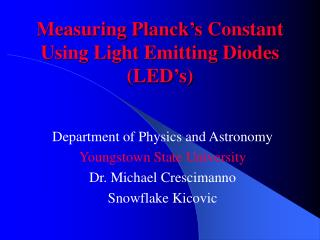DownloadDownload PresentationMeasuring Planck’s Constant Using Light Emitting Diodes (LED’s)

# Measuring Planck’s Constant Using Light Emitting Diodes (LED’s)

Télécharger la présentation## Measuring Planck’s Constant Using Light Emitting Diodes (LED’s)

- - - - - - - - - - - - - - - - - - - - - - - - - - - E N D - - - - - - - - - - - - - - - - - - - - - - - - - - -
##### Presentation Transcript

1. Measuring Planck’s Constant Using Light Emitting Diodes (LED’s) Department of Physics and Astronomy Youngstown State University Dr. Michael Crescimanno Snowflake Kicovic

2. Purpose • To find an essentially simple, straightforward method for deriving Planck’s constant using a device that we can build. • This device has to be build easily. It should be durable and feasible. • The results yielded should give an accurate value for Planck’s constant. • This method, depending on the results, can then be used in an entry level physics lab, such as that of a high school physics lab.

3. Planck’s Constant • 1900, Max Planck proposed discrete behavior for an object of subatomic dimensions - Planck’s constant h - the natural unit of action 6.626 x 10-34 J-s, or kgm2/s • It also represents angular momentum. • 1905, Einstein stated that electromagnetic radiation is localized in photons with frequency f and energy: E = hf • 1913, Niels Bohr extended idea to electron existing between states of discrete energy. Transitions are accompanied by absorption or emission of photons with f = E/h.

4. The Photoelectric Effect • 1902 it was proven that the KEmax of an electron is independent of intensity of light ray and dependent on the frequency f. • 1905, Einstein formed a fundamental theory where light is composed of photons = energy quanta. • Electrons are ejected (with great velocity from the atom) by the E of the photon. • Each light quantum consists of an amount of E = hf

5. Light Emitting Diodes • Light Emitting Diodes have p-n junctions where voltage yields a flow of current. The carriers (electrons and holes) are injected across the junction producing light.

6. Procedure • We first build the device, approximately taking 15 minutes. The device consists of 5 different colored LED’s, a 6 volt battery pack, a potentiometer, an on/off switch, a 330W resistor, a loose set of black and red wire, and a wire with an alligator clip. • The apparatus is turned on. • The alligator clip is attached to a LED lead.

7. Procedure (cont’d) • The loose wires (black and red) are connected to a Multimeter (which reads the voltage across the LED). • Turning the room lights off, we vary the voltage (with the potentiometer) to see the max voltage before shutoff of the LED. • We record the value. • After, we turn the potentiometer back to maximum, and we measure the wavelength of each diode with a spectrometer.

8. Apparatus

9. Circuit Diagram

10. The Setup

11. Blue Diode

12. Green Diode

13. Orange Diode

14. Large Red Diode

15. Small Red Diode

16. Data DiodeVoltage (V)Wavelenghts (l) Blue 2.196 640 Green 1.536 695 Orange 1.507 695 Large Red 1.530 700 Small Red 1.287 680

17. Experimental Results • From before E = hf, therefore, we used the formula h = (e V l) /c • <h> = 5.84 x 10-34 • h = (5.84 +s) x 10-34 • s = [ (1/n)Sin (hi – h)2]-1 • s = 1.05

18. Conclusion • Being that the s was 1.05, it is evident that the errors in the experiment were random rather than systematic. • This goes to show that this experiment is very effective and efficient, while at the same time being very simplistic. • These conclusions therefore exhibit the perfect characteristics for an entry level physics course while making it an interesting and EASY method for obtaining one of nature’s constants.

19. Questions?